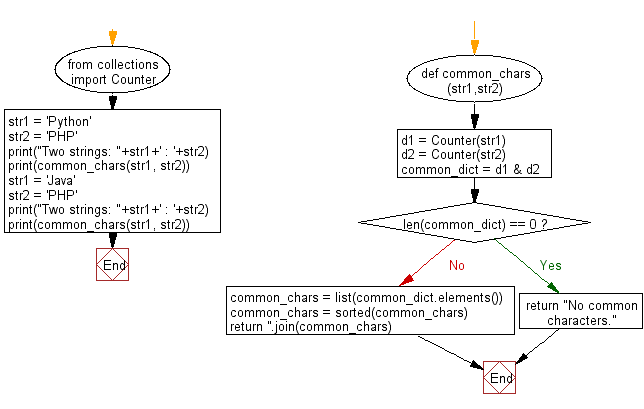﻿ Python: Find all the common characters in lexicographical order from two given lower case strings - w3resource# Python: Find all the common characters in lexicographical order from two given lower case strings

## Python String: Exercise-65 with Solution

Write a Python program to find all the common characters in lexicographical order from two given lower case strings. If there are no common letters print “No common characters”.

Sample Solution:-

Python Code:

``````from collections import Counter
def common_chars(str1,str2):
d1 = Counter(str1)
d2 = Counter(str2)
common_dict = d1 & d2
if len(common_dict) == 0:
return "No common characters."

# list of common elements
common_chars = list(common_dict.elements())
common_chars = sorted(common_chars)

return ''.join(common_chars)

str1 = 'Python'
str2 = 'PHP'
print("Two strings: "+str1+' : '+str2)
print(common_chars(str1, str2))
str1 = 'Java'
str2 = 'PHP'
print("Two strings: "+str1+' : '+str2)
print(common_chars(str1, str2))
```
```

Sample Output:

```Two strings: Python : PHP
P
Two strings: Java : PHP
No common characters.
```

Pictorial Presentation:Flowchart:## Visualize Python code execution:

The following tool visualize what the computer is doing step-by-step as it executes the said program:

Python Code Editor:

Have another way to solve this solution? Contribute your code (and comments) through Disqus.

What is the difficulty level of this exercise?

Test your Programming skills with w3resource's quiz.

﻿

## Python: Tips of the Day

Check if a given key already exists in a dictionary:

In is the intended way to test for the existence of a key in a dict.

```d = {"key1": 10, "key2": 23}

if "key1" in d:
print("this will execute")

if "nonexistent key" in d:
print("this will not")
```

If you wanted a default, you can always use dict.get():

```d = dict()

for i in range(100):
key = i % 10
d[key] = d.get(key, 0) + 1
```

and if you wanted to always ensure a default value for any key you can either use dict.setdefault() repeatedly or defaultdict from the collections module, like so:

```from collections import defaultdict

d = defaultdict(int)

for i in range(100):
d[i % 10] += 1
```

but in general, the in keyword is the best way to do it.

Ref: https://bit.ly/2XPMRyz